# Excel 2010: SUBTOTAL Function

Remembering all the functions that Excel 2010 provides is not possible, but you should know all basic functions, so that you can quickly apply them. Excel 2010 offers SUBTOTAL function that provides users with a list of functions that can be used by only entering their function number. It takes the function number of corresponding functions as an argument and location of cells to show you the subtotal of the selected data range. The SUBTOTAL function supports a total of 11 functions including AVERAGE, COUNT, MAX, MIN, SUM, VAR.S, VAR.P etc. This function prevents user from writing different subtotal functions over and over again in their spreadsheets to calculate the subtotal amount of the list. Just enter the corresponding number of the function, and it will show the required subtotal amount. In this post we will be using only four functions from the list to apply them over on the spreadsheet.

To get started, launch Excel 2010 spreadsheet on which you want to apply the SUBTOTAL function. For instance, we have included a table on which we will show you the usage of this function.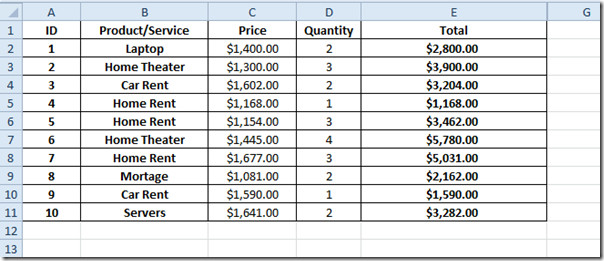The usefulness of the SUBTOTAL function is that it encapsulates a list of other functions. You just need to provide it with function_num as argument, and then reference of the location where data is residing.

The main functions it supports are; AVERAGE, COUNT, COUNTA, MAX, MIN, PRODUCT, STDEV, STDEVP, SUM, VAR, VARP.

The syntax of SUBTOTAL function is;

=SUBTOTAL(function_num, ref1, ref2…)

We will find Average, Count, Max, Sum of the Total field in the following worksheet.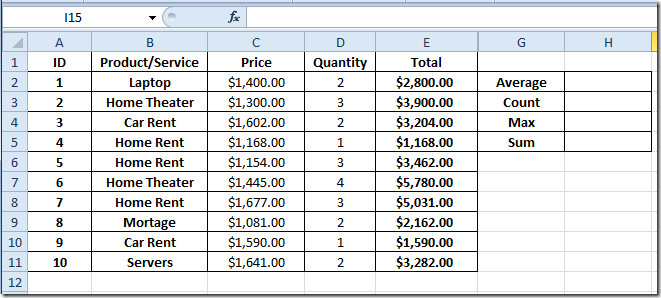In Average cell we will be writing formula as;

=SUBTOTAL(1,E2:E11)

It automatically shows the list of functions with their respective numbers. As the number assigned to AVERAGE function is 1, so we will enter 1 for finding out the average. The E2:E11 is the range of cells containing Total values.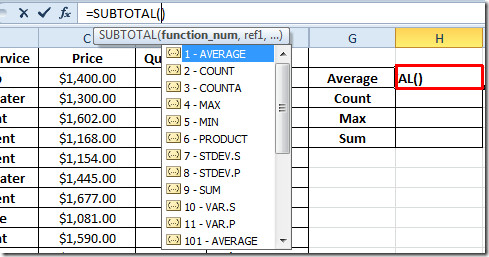It will yield average of the values present in Total field, as shown in the screen shot below.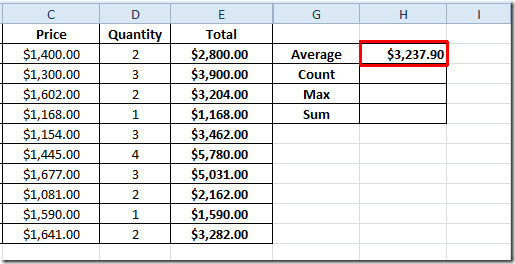Now we will find out the results from the rest of the functions;

Count  =SUBTOTAL(2,E2:E11)

Max   =SUBTOTAL(4,E2:E11)

Sum   =SUBTOTAL(9,E2:E11)

The result of the all the functions we wanted to evaluate is shown in the table below. It must be noted that if you want to calculate the different subtotal amount of a list, provide it with the correct data range for all functions, as it will not show you an error for different data ranges.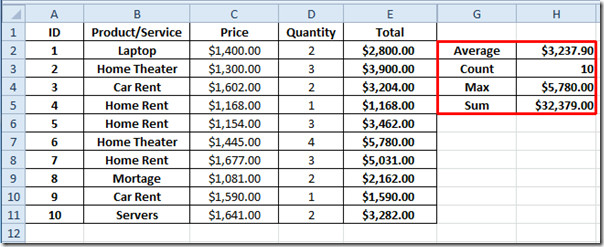You can also check out previously reviewed Excel functions; CONCATENATE, DOLLAR, SUMPRODUCT, SUMIF, COUNTIF, VLOOKUP, HLOOKUP ,PMT, and LEN.

### 1 Comment

1.alok says:

hi,
what is the Keyboard Shortcut 4 Moving Btwn Subtotals Groups

This site uses Akismet to reduce spam. Learn how your comment data is processed.